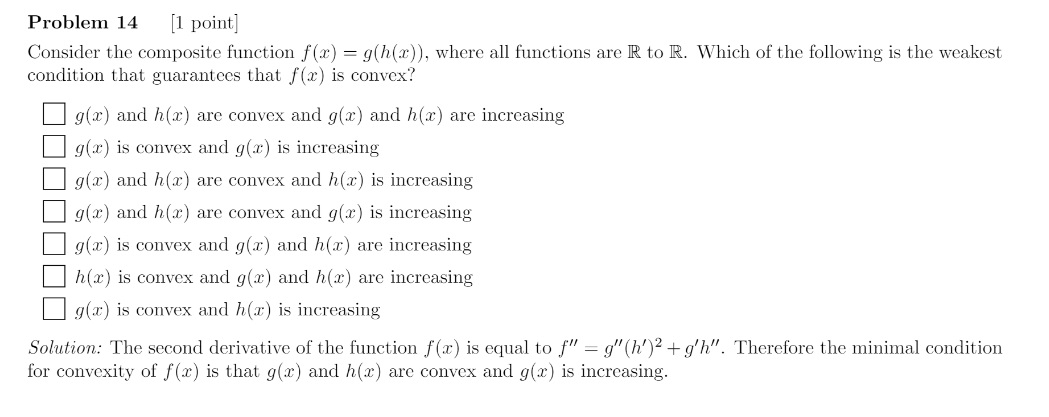### Convexity of g(h(x)) exam 2017

Hi,Here I think that the derivative should be

f''(x) = g''o h(x) h'(x)² + h''(x) g' o h(x)

How can we deduce from this that g is increasing and h&g are convex ?

I know that we want f''(x) >= 0 however I don't know how we can deduce from this solution equation g'' >= 0, h''(x) >= 0 and g'(x) >= 0 ?
We could have h''(x) < 0 and g'(h(x)) < 0 and still have f''(x) > 0.

Top comment

Yes what is written in the solution is meant to mean what you wrote : $$f''(x) = g''o h(x) h'(x)² + h''(x) g' o h(x)$$.

So now if g and h are convex then $$g''\geq 0$$ and $$h''\geq 0$$. But we we would still need to have $$g'\geq 0$$ i.e. g to be increasing to have $$f''\geq 0$$.

Yes, you are right if h is concave and g is decreasing and convex then f would be convex too, but it is a multiple choice question and the answer is limiited to the choices.

Anyway, using second derivatives and even first derivatives is quite strong, you can arrive at the conclusions above by only using the definition of convexity.

Page 1 of 1